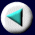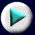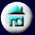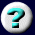11. Conservation of Mass,       Charge, and EnergyMass and Energy: Nuclear Reactions
 (Compare this energy with the 83 kcal mole-1 required to break carbon-carbon bonds in chemical reactions.) Protons and neutrons collectively are known as nuclear particles or nucleons. The number of nucleons in the nucleus is the sum of the number of protons and neutrons and, as was mentioned in Chapter 2, is known as the mass number of the nucleus. Every atomic nucleus is lighter than the sum of the masses of the nucleons from which it is built, and this mass loss corresponds to the binding energy of the nucleus. The relative stability of two nuclei with different numbers of nucleons can be assessed by comparing their mass loss per nucleon. Example: What is the mass loss per nucleon for the helium atom? Solution: The total mass loss is 0.0305 amu, and since the nucleus has four nucleons, the mass loss per nucleon is 0.00763 amu.
Page 23 of 37